# Visualize bundles and metrics on bundles#

First, let’s download some available datasets. Here we are using a dataset which provides metrics and bundles.

```import numpy as np
from dipy.tracking.streamline import length, transform_streamlines

from fury import actor, window

interactive = False  # set to True to show the interactive display window

fetch_bundles_2_subjects()
subj_id='subj_1', metrics=['fa'], bundles=['cg.left', 'cst.right']
)
```

Store fractional anisotropy.

```fa = dix['fa']
```

Store grid to world transformation matrix.

```affine = dix['affine']
```

Store the cingulum bundle. A bundle is a list of streamlines.

```bundle = dix['cg.left']
```

It happened that this bundle is in world coordinates and therefore we need to transform it into native image coordinates so that it is in the same coordinate space as the `fa` image.

```bundle_native = transform_streamlines(bundle, np.linalg.inv(affine))
```

## Show every streamline with an orientation color#

This is the default option when you are using `line` or `streamtube`.

```scene = window.Scene()

stream_actor = actor.line(bundle_native)

scene.set_camera(
position=(-176.42, 118.52, 128.20),
focal_point=(113.30, 128.31, 76.56),
view_up=(0.18, 0.00, 0.98),
)

if interactive:
window.show(scene, size=(600, 600), reset_camera=False)

window.record(scene, out_path='bundle1.png', size=(600, 600))
```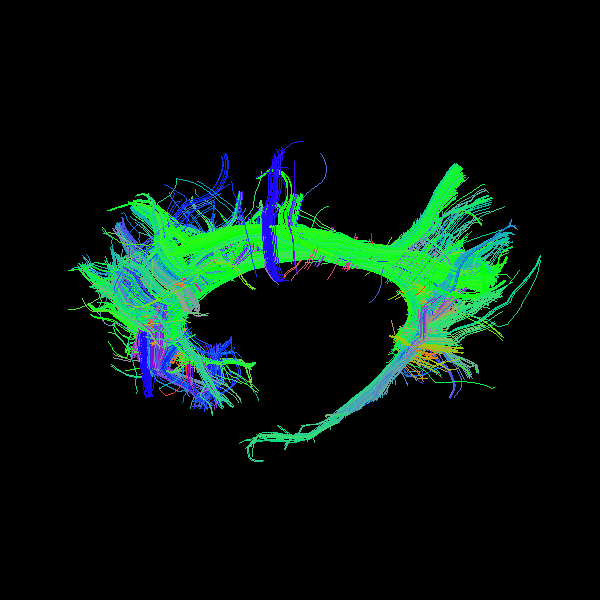You may wonder how we knew how to set the camera. This is very easy. You just need to run `window.show` once see how you want to see the object and then close the window and call the `camera_info` method which prints the position, focal point and view up vectors of the camera.

```scene.camera_info()
```
```# Active Camera
Position (-237.76, 115.97, 138.55)
Focal Point (112.80, 127.81, 76.06)
View Up (0.18, 0.00, 0.98)
```

## Show every point with a value from a volume with default colormap#

Here we will need to input the `fa` map in `streamtube` or `line`.

```scene.clear()
stream_actor2 = actor.line(bundle_native, fa, linewidth=0.1)
```

We can also show the scalar bar.

```bar = actor.scalar_bar()

if interactive:
window.show(scene, size=(600, 600), reset_camera=False)

window.record(scene, out_path='bundle2.png', size=(600, 600))
```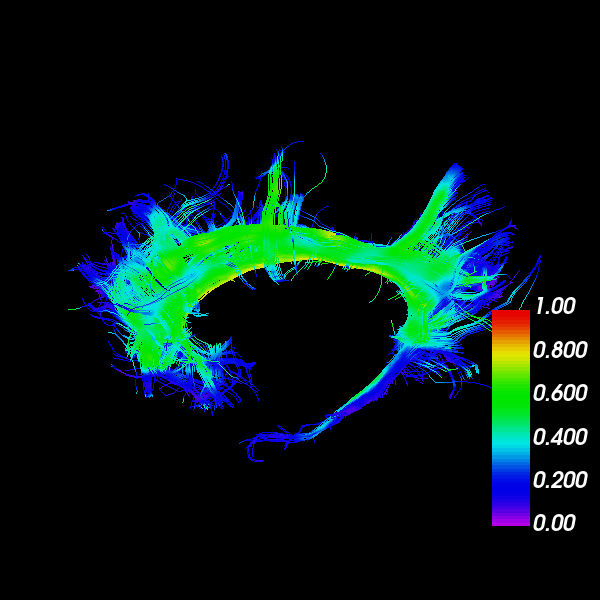## Show every point with a value from a volume with your colormap#

Here we will need to input the `fa` map in `streamtube`

```scene.clear()

hue = (0.0, 0.0)  # red only
saturation = (0.0, 1.0)  # white to red

lut_cmap = actor.colormap_lookup_table(hue_range=hue, saturation_range=saturation)

stream_actor3 = actor.line(bundle_native, fa, linewidth=0.1, lookup_colormap=lut_cmap)
bar2 = actor.scalar_bar(lut_cmap)

if interactive:
window.show(scene, size=(600, 600), reset_camera=False)

window.record(scene, out_path='bundle3.png', size=(600, 600))
```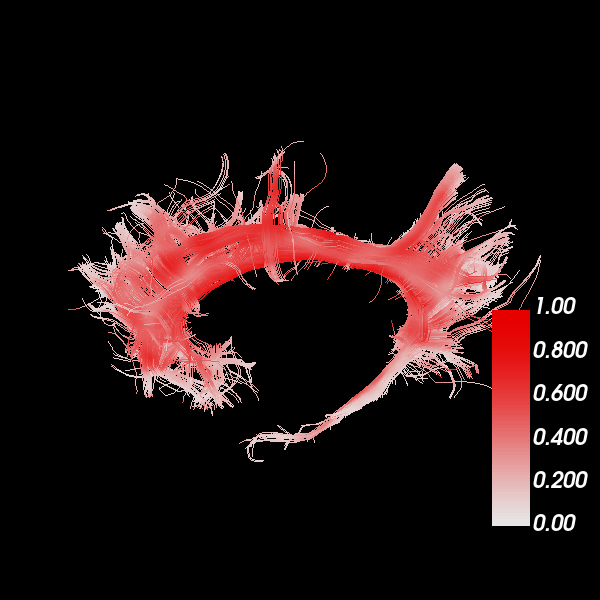## Show every bundle with a specific color#

You can have a bundle with a specific color. In this example, we are choosing orange.

```scene.clear()
stream_actor4 = actor.line(bundle_native, (1.0, 0.5, 0), linewidth=0.1)

if interactive:
window.show(scene, size=(600, 600), reset_camera=False)

window.record(scene, out_path='bundle4.png', size=(600, 600))
```## Show every streamline of a bundle with a different color#

Let’s make a colormap where every streamline of the bundle is colored by its length.

```scene.clear()

lengths = length(bundle_native)

hue = (0.5, 0.5)  # blue only
saturation = (0.0, 1.0)  # black to white

lut_cmap = actor.colormap_lookup_table(
scale_range=(lengths.min(), lengths.max()),
hue_range=hue,
saturation_range=saturation,
)

stream_actor5 = actor.line(
bundle_native, lengths, linewidth=0.1, lookup_colormap=lut_cmap
)

bar3 = actor.scalar_bar(lut_cmap)

if interactive:
window.show(scene, size=(600, 600), reset_camera=False)

window.record(scene, out_path='bundle5.png', size=(600, 600))
```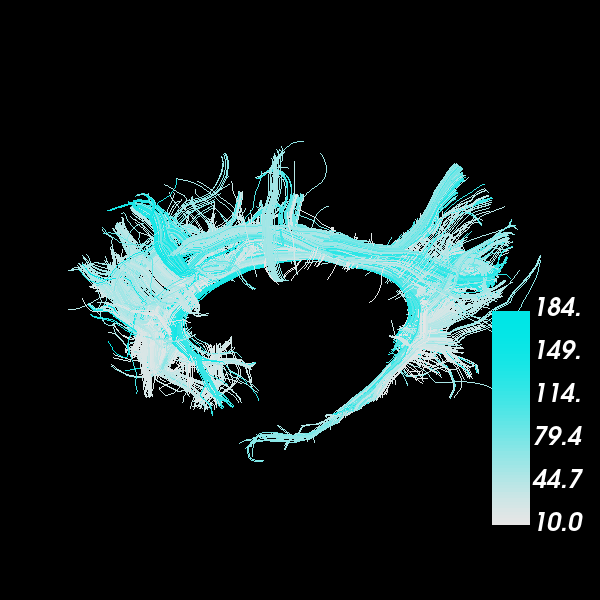## Show every point of every streamline with a different color#

In this case in which we want to have a color per point and per streamline, we can create a list of the colors to correspond to the list of streamlines (bundles). Here in `colors` we will insert some random RGB colors.

```scene.clear()

colors = [np.random.rand(*streamline.shape) for streamline in bundle_native]

stream_actor6 = actor.line(bundle_native, np.vstack(colors), linewidth=0.2)

if interactive:
window.show(scene, size=(600, 600), reset_camera=False)

window.record(scene, out_path='bundle6.png', size=(600, 600))
```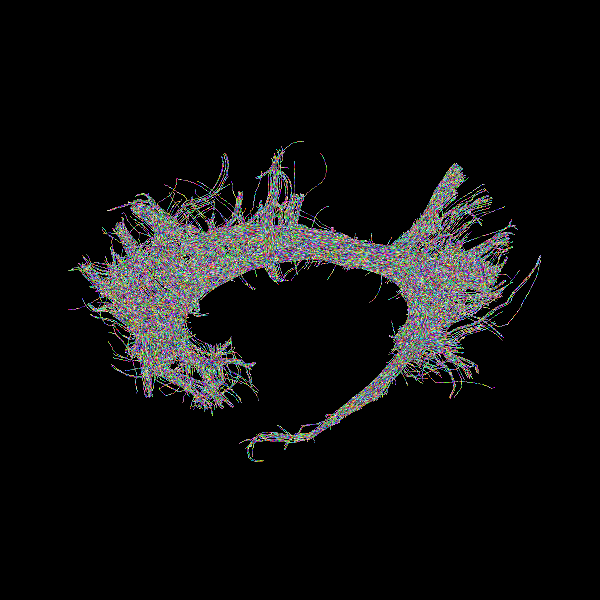## Add depth cues to streamline rendering#

By default, lines are drawn with the same width on the screen, regardless of their distance from the camera. To increase realism, we can enable `depth_cue` to make the lines shrink with distance from the camera. We will return to the default color scheme from the first example. Note that `depth_cue` works best for `linewidth` <= 1.

```scene.clear()

stream_actor7 = actor.line(bundle_native, linewidth=0.5, depth_cue=True)

if interactive:
window.show(scene, size=(600, 600), reset_camera=False)

window.record(scene, out_path='bundle7.png', size=(600, 600))
```## Render streamlines as fake tubes#

We can simulate the look of streamtubes by adding shading to streamlines with `fake_tube`. Note that `fake_tube` requires `linewidth` > 1.

```scene.clear()

stream_actor8 = actor.line(bundle_native, linewidth=3, fake_tube=True)

if interactive:
window.show(scene, size=(600, 600), reset_camera=False)

window.record(scene, out_path='bundle8.png', size=(600, 600))
```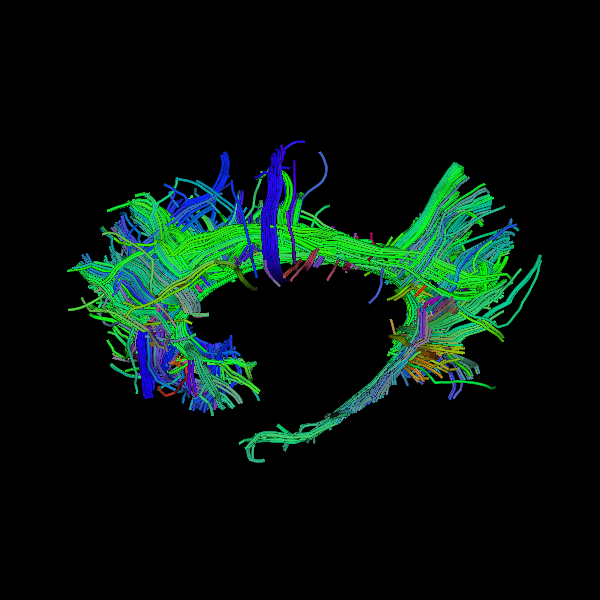## Combine depth cues with fake tubes#

It is possible to fully simulate streamtubes by enabling both `depth_cue` and `fake_tube`. However, it can be challenging to choose a `linewidth` that demonstrates both techniques well.

```scene.clear()

stream_actor9 = actor.line(bundle_native, linewidth=3, depth_cue=True, fake_tube=True)

if interactive:
window.show(scene, size=(600, 600), reset_camera=False)

window.record(scene, out_path='bundle9.png', size=(600, 600))
```## Render streamlines as tubes#

For yet more realism, we can use `streamtube`. Note that this actor generates much more geometry than `line`, so it is more computationally expensive. For large datasets, it may be better to approximate tubes using the methods described above.

```scene.clear()

stream_actor10 = actor.streamtube(bundle_native, linewidth=0.5)